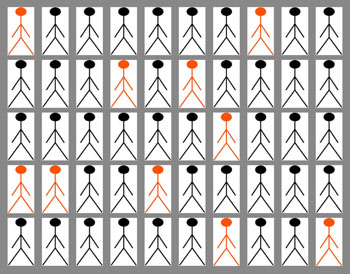# 15.3.1  Simple random sampling

As the name suggests, simple random sampling is the simplest method of probability sampling. It means within a particular study population everyone has an equal chance of inclusion in the sample. It is considered ‘fair’ and therefore allows findings to be generalised to the whole population from which the sample was taken. It is sometimes called the ‘lottery method’ and is illustrated in Figure 15.1.Figure 15.1  An example of simple random sampling of 10 subjects, represented by the red ‘stickmen’, selected at random from a total of 50 subjects using a lottery method. (Diagram: Jessica Aumann)

To use the simple random sampling method, it is necessary to have lists of all elements of the population to be studied. Therefore, to select a simple random sample you need to:

• Make or search for an existing named or numbered list of all the members in the study population from which you want to take a sample.
• Decide on the size of the sample you need (this will be discussed in Section 15.6).
• Select the required number of subjects (also known as ‘sampling units’) using a lottery method so everyone has an equal chance of being selected. Case Study 15.1 illustrates one way of doing this.

## Case Study 15.1  Selecting a simple random sample of students

A simple random sample of 25 students is to be selected from a school of 500 students (Figure 15.2). Using a list of all 500 students, each student is given a number (1 to 500), and these numbers are written on small pieces of paper. All the 500 papers are put in a box, after which the box is shaken vigorously to ensure randomisation. Then, 25 papers are taken out of the box, and the numbers are recorded. The students belonging to these numbers will constitute the simple random sample.Figure 15.2  Random sampling of school students will provide a representative sample. (Photo: Pam Furniss)

15.3  Probability sampling methods

15.3.2  Systematic sampling